# High School Chemistry : Help with Gibb's Free Energy

## Example Questions

### Example Question #1 : Help With Gibb's Free Energy

What set of conditions always results in a spontaneous reaction?

Negative enthalpy change and negative entropy change

Positive enthalpy change and negative entropy change

Positive enthalpy change and positive entropy change

Negative enthalpy change and positive entropy change

Negative enthalpy change and positive entropy change

Explanation:

The spontaneity of a reaction can be determined using the Gibb's free energy equation: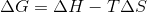In this formula,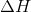is enthalpy,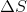is entropy, and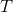is the temperature in Kelvin. In order for a reaction to be spontaneous, Gibb's free energy must be negative. Looking at the equation, we can see that the value for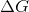will ALWAYS be negative if enthalpy is negative and entropy is positive.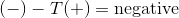These are the requirements for a reaction that is always spontaneous, regardless of temperature,

### Example Question #2 : Help With Gibb's Free Energy

A reaction has an enthalpy change of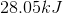and an entropy change of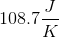.

At what temperature range will the reaction be spontaneous?

Lower than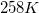Greater thanLower than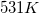Higher thanGreater thanExplanation:

The first thing we need to do in order to solve this problem is determine at what temperatureis equal to zero. Whenis equal to zero, the reaction is at equilibrium and will not go one way or the other. Using the Gibb's free energy equation, we can determine the temperature where the reaction is at equilibrium.The question tells us the enthalpy and entropy values, allowing us to solve for the equilibrium temperature. Remember to convert the enthalpy from kilojoules to Joules.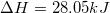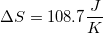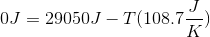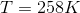At, the reaction is at equilibrium. In order to make the reaction spontaneous, do we need to raise or lower from this temperature? Notice how entropy in this case is positive. By increasing temperature, the negative portion of the equation will become larger, and will result in a negative value. This means that the above reaction is spontaneous at temperatures higher than. You can check you work by solving for the Gibb's free energy at a higher temperature.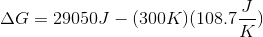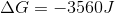Since the value is negative, the reaction is spontaneous at this higher temperature.

### Example Question #531 : High School Chemistry

Predict the spontaneity of a reaction at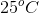with an enthalpy change of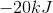and an entropy change of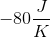.

We must know the moles of gas to predict spontaneity at this temperature

Spontaneous

Reaction is at equilibrium

Nonspontaneous

Nonspontaneous

Explanation:

Given the above conditions, we can use the Gibb's free energy equation in order to determine the reaction's spontaneity.Convert the temperature to Kelvin and the enthalpy to Joules.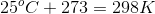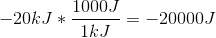Use the given values to solve for the Gibb's free energy.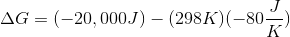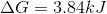Sinceis positive at these conditions, we can conclude that the reaction is nonspontaneous and will not take place.

### Example Question #2 : Help With Gibb's Free Energy

The change in Gibb's free energy of a spontaneous reaction is __________.

only less than zero at low temperatures

equal to zero

only greater than zero at high temperatures

less than zero

greater than zero

less than zero

Explanation:

The sign of the change in Gibb's free energy,tells us whether a reaction is spontaneous or not (whether the reaction requires the net input of energy or not). For a spontaneous reaction, thevalue is always less than zero. This is because the free energy of the products is less than that of the reactants. This indicates that there is a net release of energy, as opposed to a net energy consumption. When the products are at a lower energy than the reactants, the reaction can proceed spontaneously, and is known as exothermic.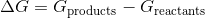### Example Question #1 : Help With Gibb's Free Energy

Which of the following best describes the change in Gibbs free energy when a sample of ice is melting at a temperature of 21oC?

Sometimes positive

Always negative

Sometimes negative

Always positive

Positive or negative depending on the volume of the ice

Always negative

Explanation:

Gibbs free energy is used to determine if a reaction will occur spontaneously. If the Gibbs free energy is negative for the given process, then the reaction will occur spontaneously.

In the given scenario, ice will always melt spontaneously when the temperature is above 0oC. As a result, we can state that the Gibbs free energy will always be negative for this process.

### Example Question #6 : Help With Gibb's Free Energy

If a process is endothermic and involves a decrease in entropy, when will it be spontaneous?

At low temperatures

In zero gravity

At high temperatures

Never

Never

Explanation:.

If a process is endothermic,is positive.

If a process involves a decrease in entropy,is negative.

Under these conditions, the only waycan be negative is ifis below absolute zero, which is impossible. Catalysts do not effect, rather they only affect reaction kinetics, not thermodynamics.

### All High School Chemistry Resources